Constructing angles using protractor

Practical Geometry
Serial order wise

Here, we will draw right angle i.e. 45° using protractor.

To draw 45° using compass, please check Ex 11.1, 2 of Class 9 NCERT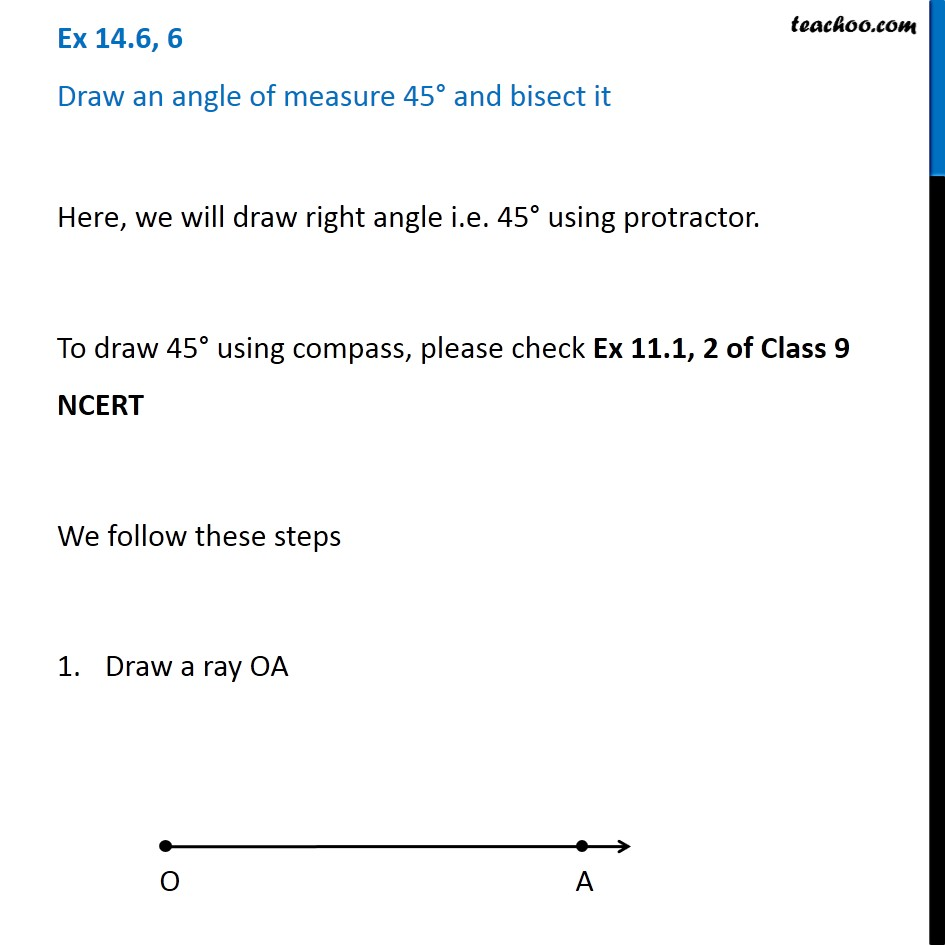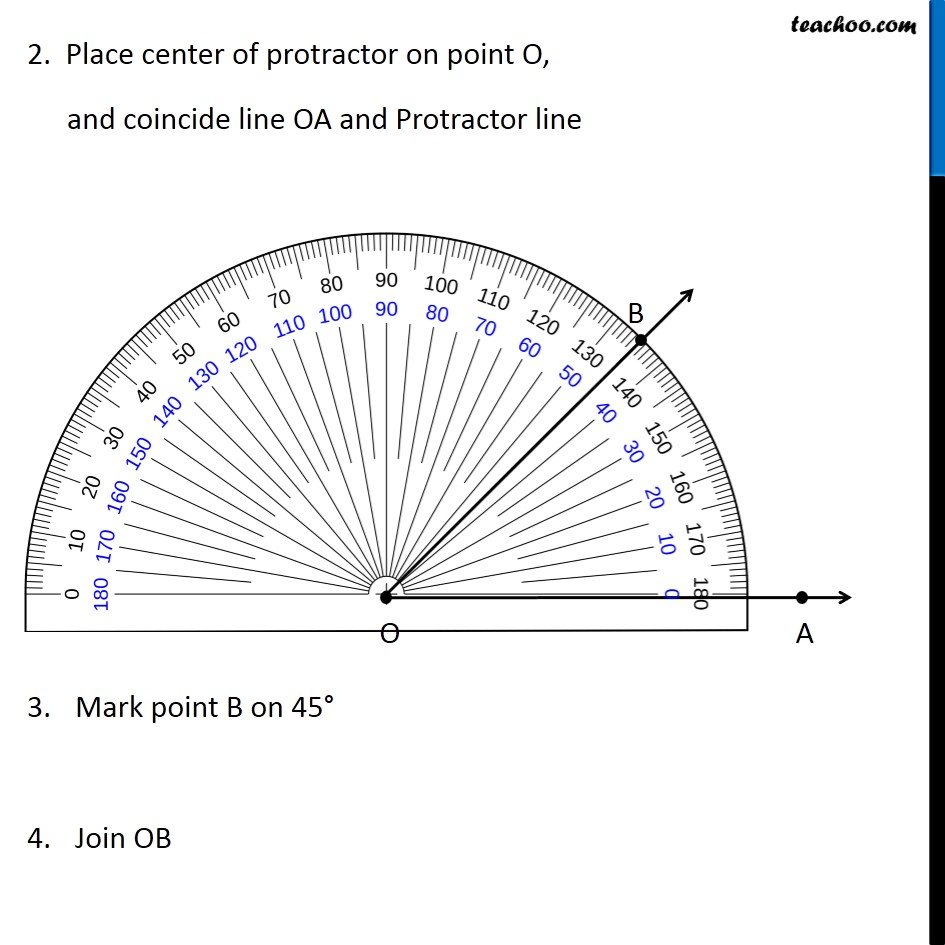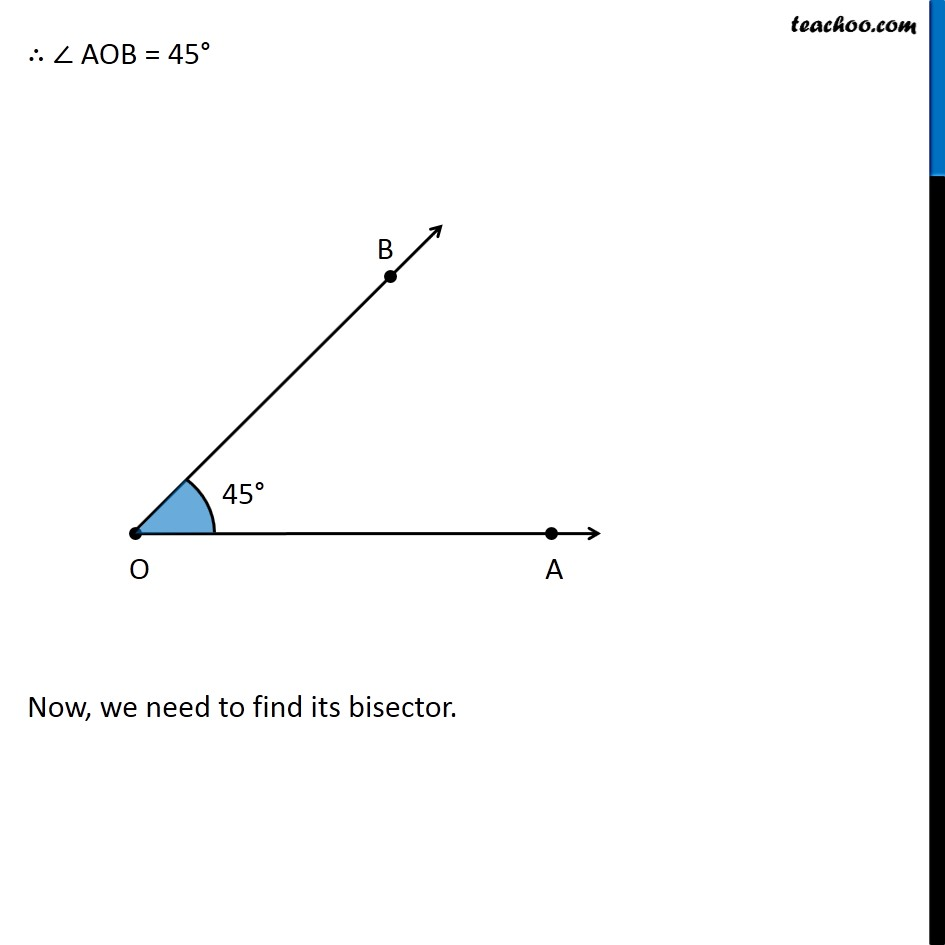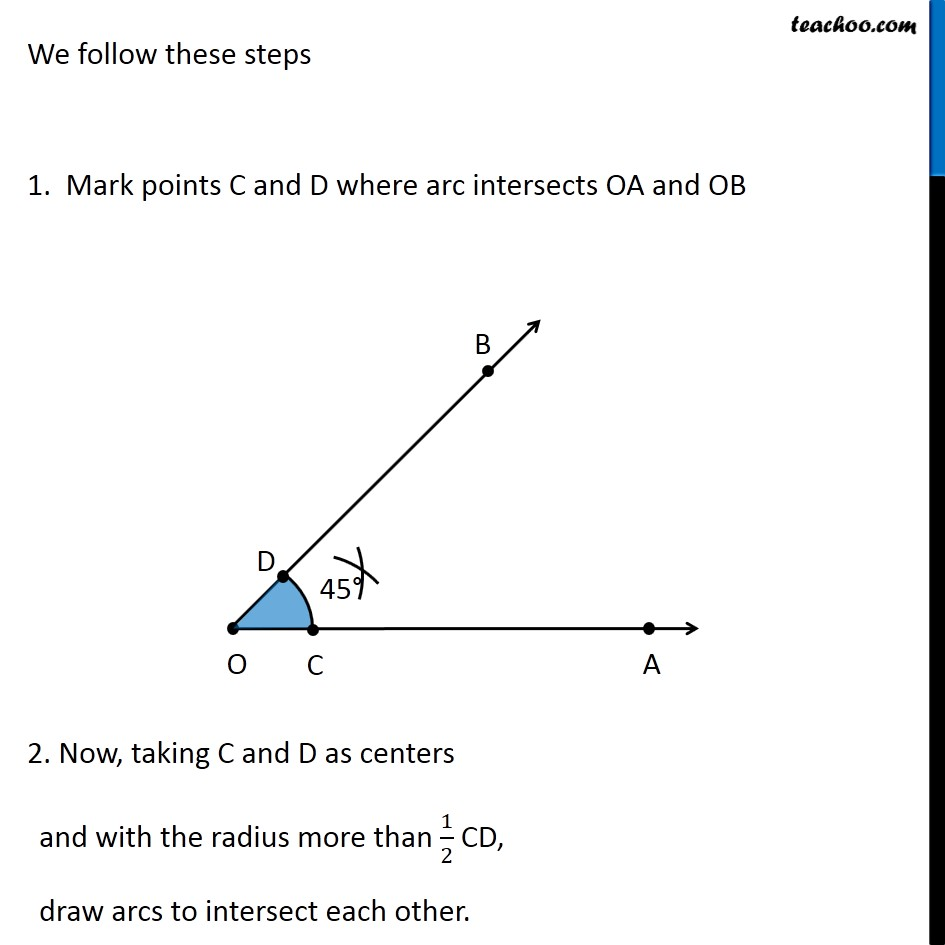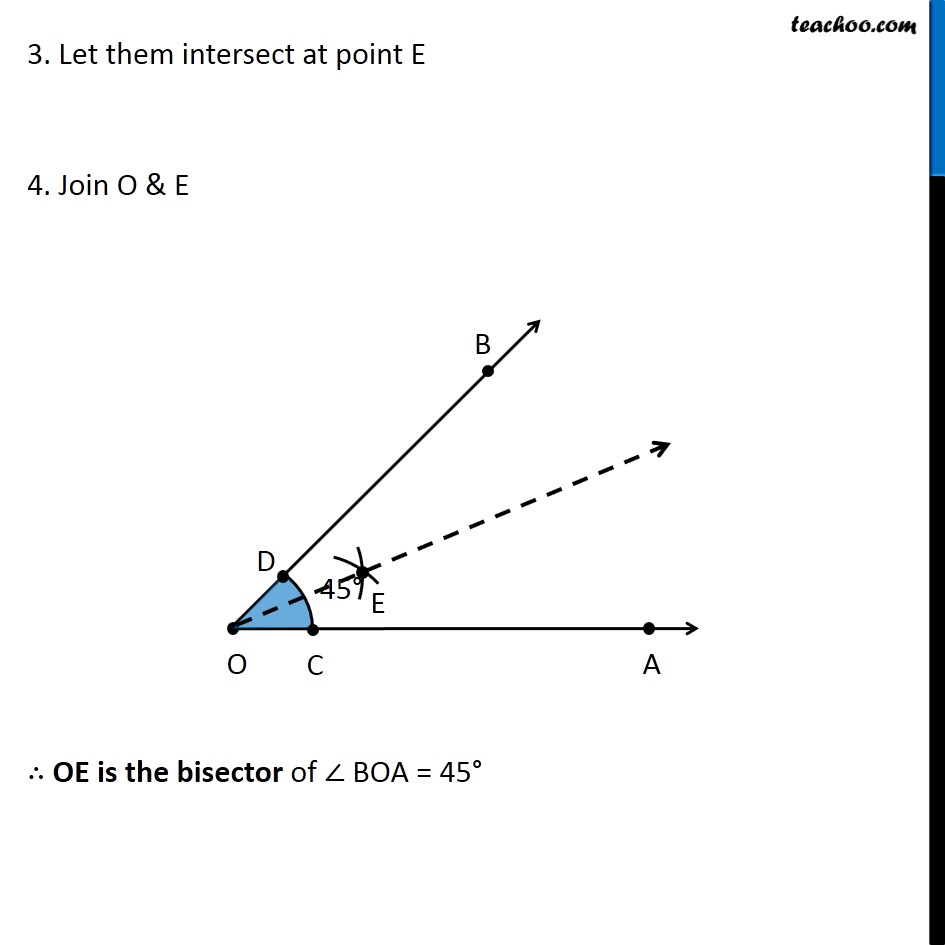Learn in your speed, with individual attention - Teachoo Maths 1-on-1 Class

### Transcript

Question 6 Draw an angle of measure 45° and bisect it Here, we will draw right angle i.e. 45° using protractor. To draw 45° using compass, please check Ex 11.1, 2 of Class 9 NCERT We follow these steps Draw a ray OA 2. Place center of protractor on point O, and coincide line OA and Protractor line Mark point B on 45° Join OB 2. Place center of protractor on point O, and coincide line OA and Protractor line Mark point B on 45° Join OB ∴ ∠ AOB = 45° Now, we need to find its bisector. We follow these steps 1. Mark points C and D where arc intersects OA and OB 2. Now, taking C and D as centers and with the radius more than 1/2 CD, draw arcs to intersect each other. 3. Let them intersect at point E 4. Join O & E ∴ OE is the bisector of ∠ BOA = 45°